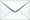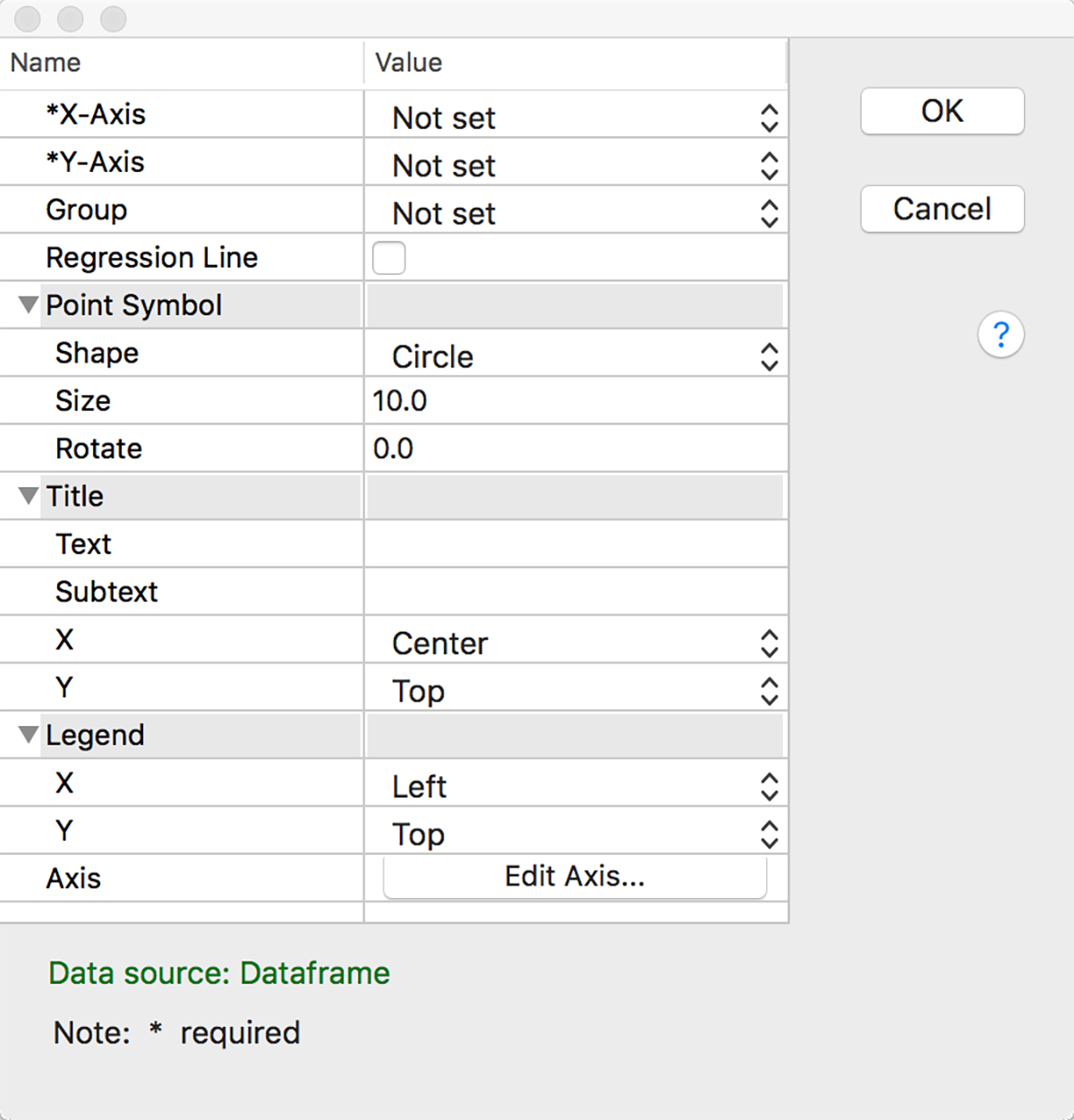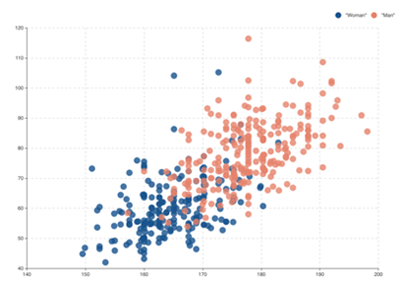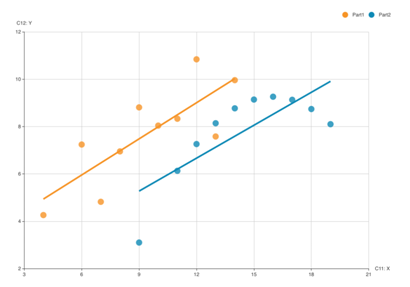Product Search

Dear customers:

take action as soon as possible.tyevolution@yahoo.com

# Scatter: Basic Scatter

Views:111times*X-Axis: Enter a column of x-values.

*Y-Axis: Enter a column of y-values.

Group: Check to display all x-y pairs as separate groups on the same graph.

Regression Line: Use to illustrate the relationship between a predictor and response variable and to see if your model fits the data. This line is a graphical representation of the mathematical regression equation.  It is plotted using the least squares method which minimizes the sum of the squared distances between the points and the fitted line.

Examples